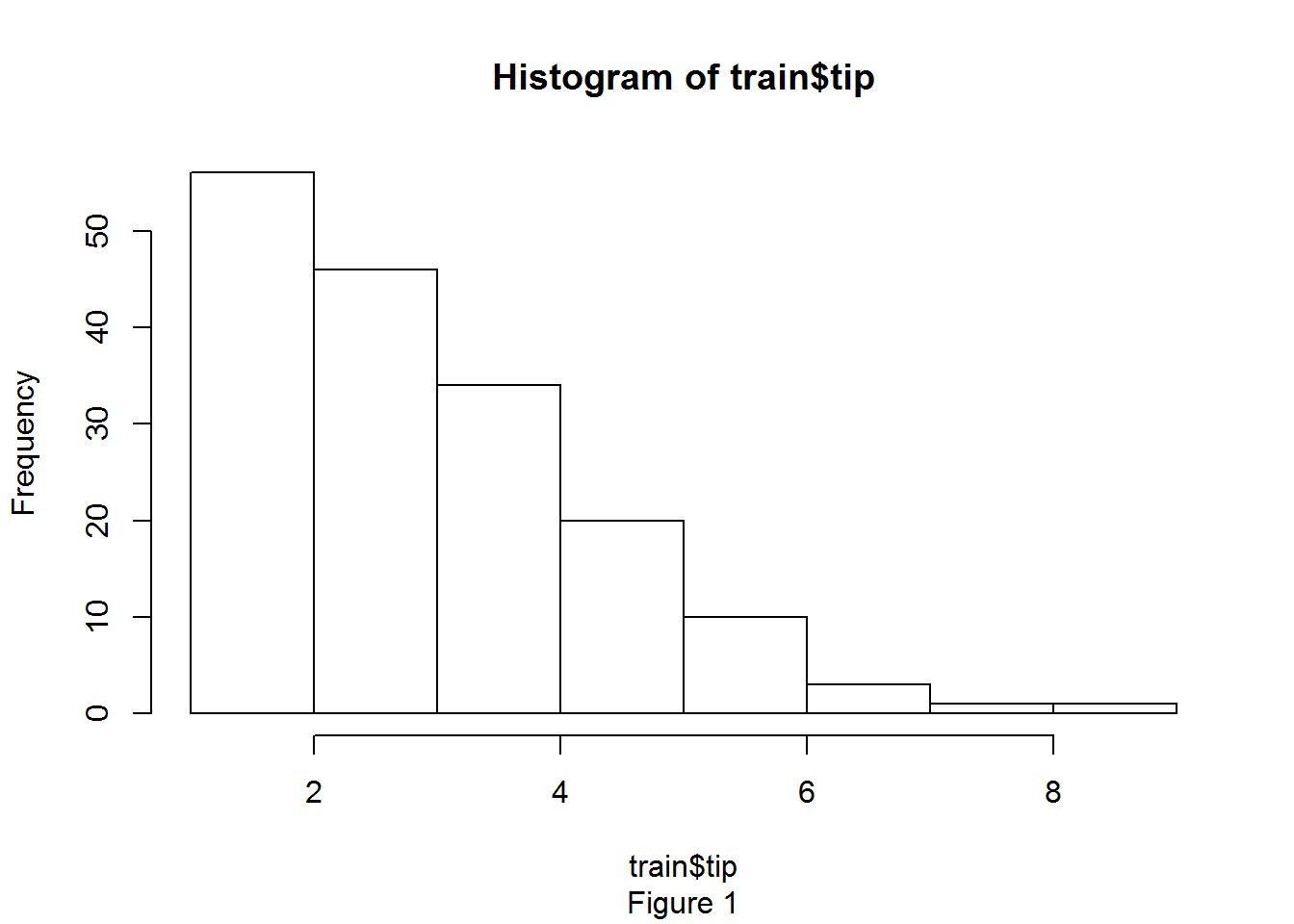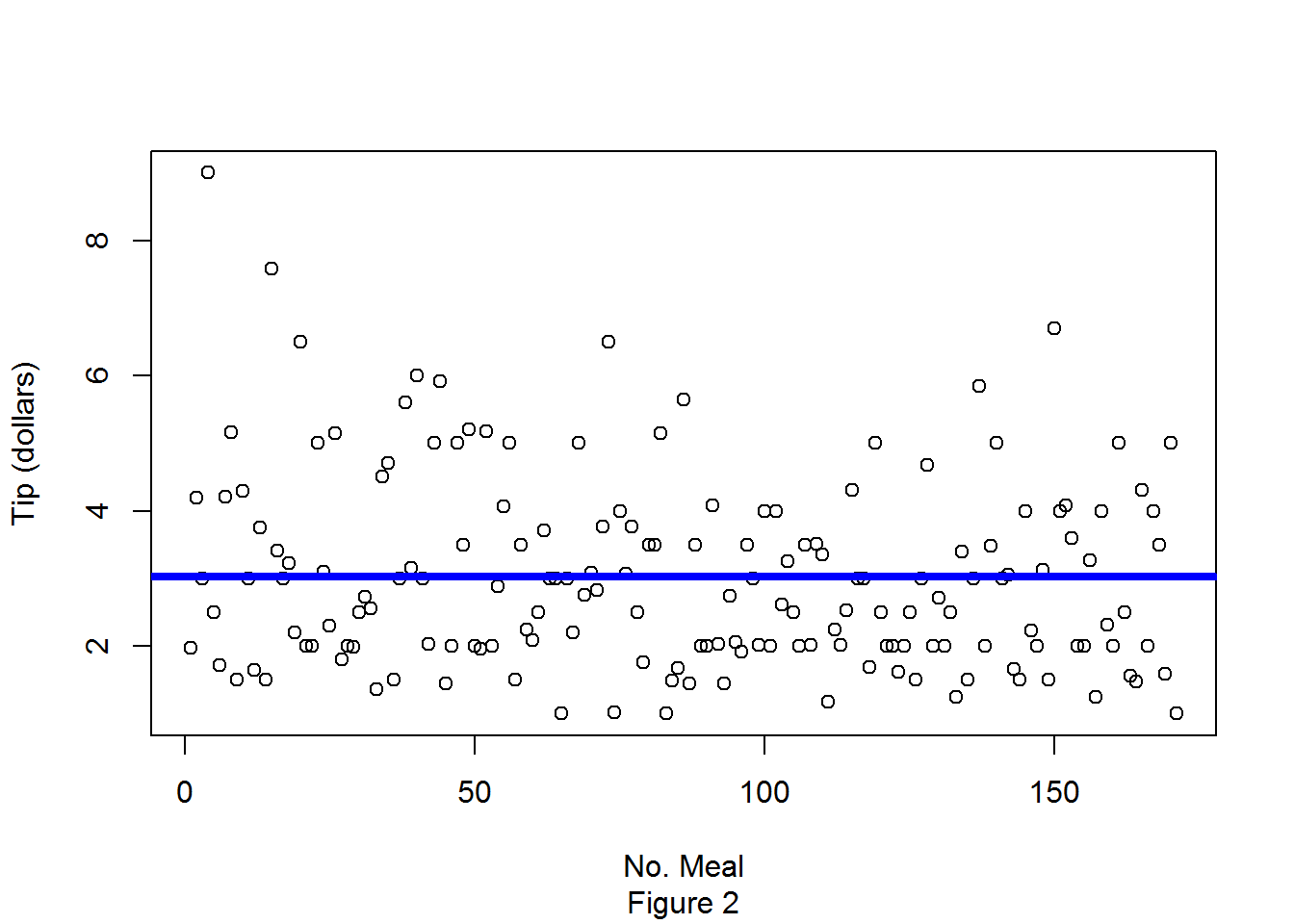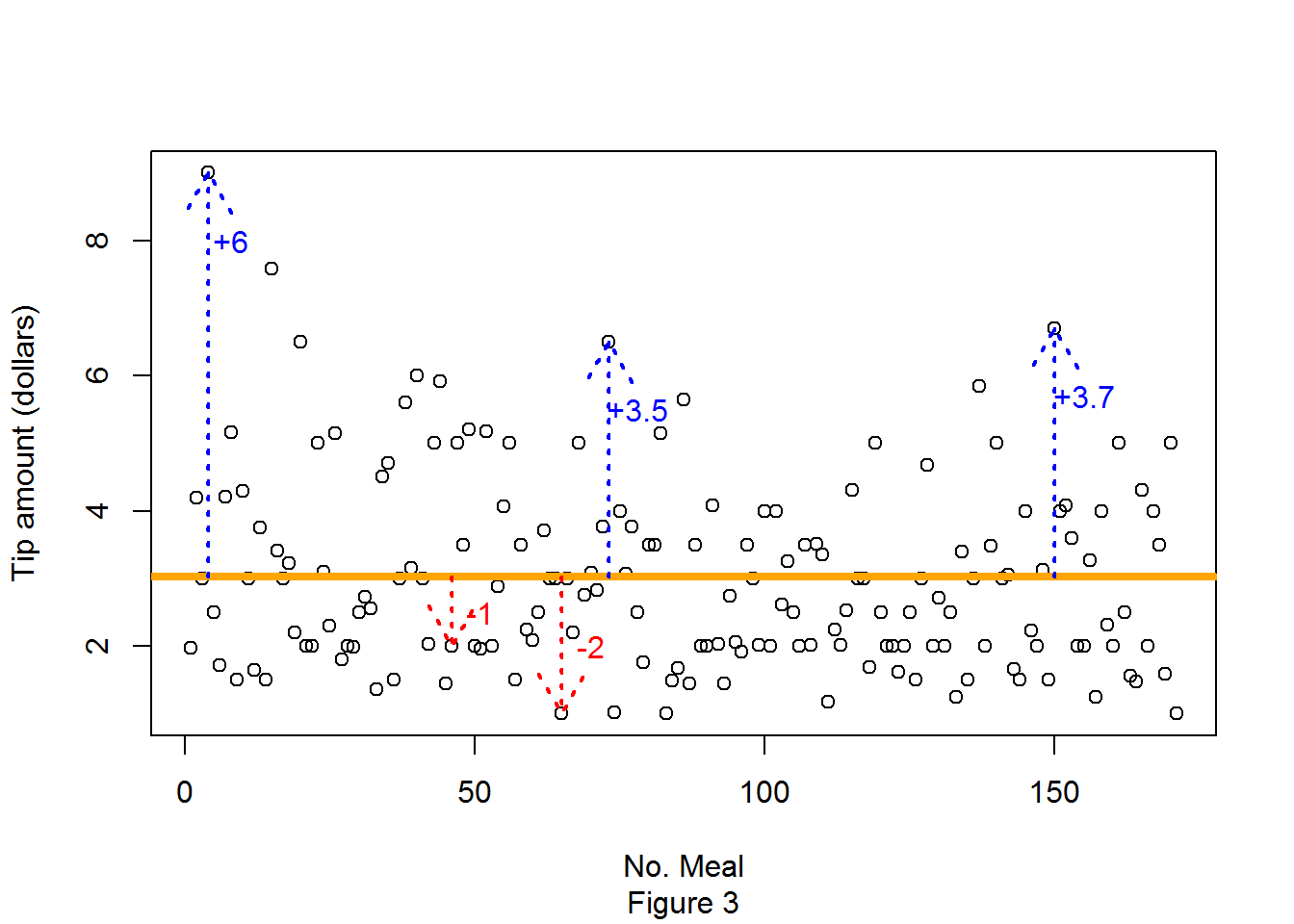## Monday, August 3, 2015

### How much to tip at a restaurant? (Part 1 of 2)

How much to tip at a restaurant? (Part 1 of 2)

## Introduction

Always wondering how much to tip at a restaurant? Today we are going to build a model to help us with this decision, in this analysis we’ll use one of the most basic statistical prediction techniques :“linear regression”. The dataset we’ll use is a record of the tips that a waiter received over a period of a few months, he recorded a total of 244 events. The data comes from the book: Practical Data Analysis: Case Studies in Business Statistics (1).

## Data Pre-processing

The dataset has 7 variables, but today we will focus on a simple linear regression model which uses only two variables:
• Bill. Dollars representing the value of a meal. This is our independent variable ( the one that helps us to predict tip).
• Tip. Dollars that the customer left by a service provided. This is our dependent variable (the one we are trying to predict). We expect that the dependent variable changes depending on the independent variable.
A very popular type of algorithms known as supervised algorithms, like linear reggresion, need to be trained (training phase) before making predictions (testing phase) . The testing set is never used for training because it should represent data not seen by the model in the training phase. In some scenarios there is a training dataset and a testing set, however in this scenario we only have a single dataset, we will hold a fraction of this data as testing set (70%) and the rest will be used as training set (30%).

## Exploratory analysis

Now let’s see how looks the data, for this we can print only the first rows of the dataset.
##   total_bill  tip
## 1      12.02 1.97
## 2      19.81 4.19
## 3      21.01 3.00
## 4      48.33 9.00
## 5      16.27 2.50
## 6      10.27 1.71
Looking directly at the dataset makes sense if the number of records or observations is very small, however even in this case where the number of observations (244) is not very large (compared with real world datasets with more than millions of observations) it is difficult to see a pattern or a trend simply by looking at a table. A good idea in this scenario with only two variables is to use a graphical representation of our data.
Then, let’s plot the size of the tip left by each customer with the help of an histogram (Figure 1), We can see in the following graph that the tips form a slope from left to right (knew has right skew distribution), this means that the clients tend to leave relatively small tips. Ex. Almost 80 clients left a tip around $2 while 1 client left a tip of around$10. We can be inclined to conclude that the clients at this restaurant are cheap or that the waiter really sucks, but a better approach could be that the bills amount are in general low, with only a few really big bills and then the tip amount varies accordingly to the size of the bill.
hist(train$tip,sub="Figure 1")## The method ### The base model The previous plot helped us to see a tendency to received small tips. Now, let’s build a scatterplot to try to better understand the tips behavior(Figure 2). The following graphic shows the Tip size left in each meal. A first attempt is to use the average of these tips. This can be equivalent to ask a waiter about the average tip that he receives and the waiter answers with the average tip without taking into account the size of the bill. The mean for the tips variable is$3. We can observe this value as the horizontal blue line in the Figure 2 , this line represents the mean of all the tips in the training set. This way of using the mean of the dependent variable to predict its own future value is used as a base model with which to compare more complex models.An important consideration in any model is to measure how well it represents the data. Our first model that only uses the average of the tips is named the base model and we want to measure how far or close away from the true tips behavior is our base model, for this we need to obtain the distance of each tip to the average tip (blue line in Figure 3), this is each bill - average bill, then we square this difference to transform negative differences into positives differences, finally the sum of this squared differences is the sum of suares total of the base model (SST). In this case the SST equals 340.91. We will use this SST as a base with which to compare a more complex model that uses the size of the bill to predict the size of the tip, then determine if it shows an improvement over a simple based model. The complet process to obtain the SST is shown in Table 1.Table 1
tips mean difference Squared
1.97 - 3.02 = -1.05 ^2 = 1.11
4.19 - 3.02 = 1.17 ^2 = 1.36
3.00 - 3.02 = -0.02 ^2 = 0.00
9.00 - 3.02 = 5.98 ^2 = 35.71
2.50 - 3.02 = -0.52 ^2 = 0.27
1.71 - 3.02 = -1.31 ^2 = 1.73
4.20 - 3.02 = 1.18 ^2 = 1.38
5.16 - 3.02 = 2.14 ^2 = 4.56
1.50 - 3.02 = -1.52 ^2 = 2.32
4.29 - 3.02 = 1.27 ^2 = 1.60
This base model is not very helpful as it only uses the mean as the prediction for all meals, no matter if the amount is really big or small. This model is too simple and assigns the same tip for every case. In the second part of this post we will build a more interesting model using the size of the bill to predict the size of the tip.
1. Peter G. Bryant and Marlene A. Smith. 1994. Practical Data Analysis: Case Studies in Business Statistics (1st ed.). McGraw-Hill Professional.